# Page rank2

25. Oct 2012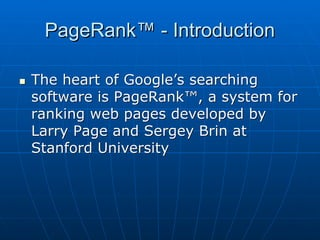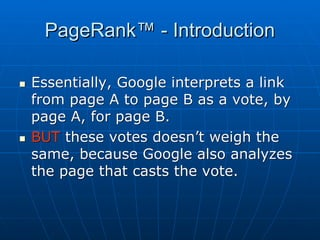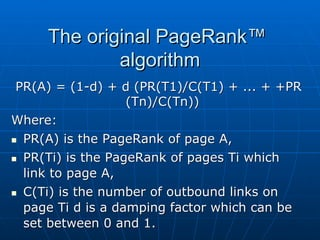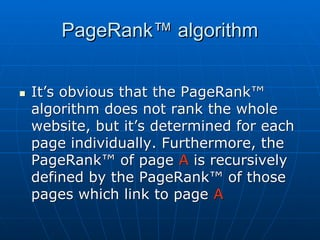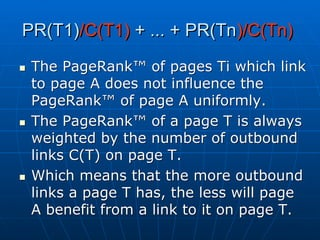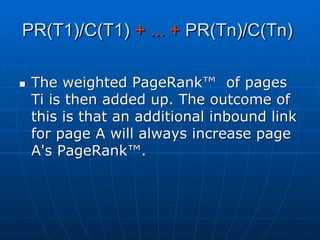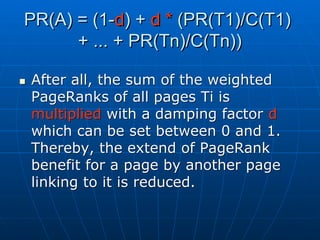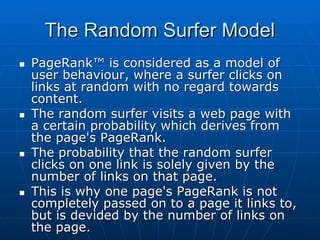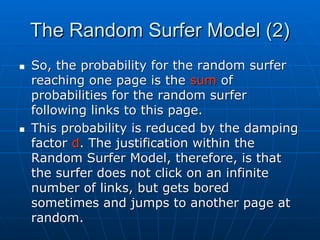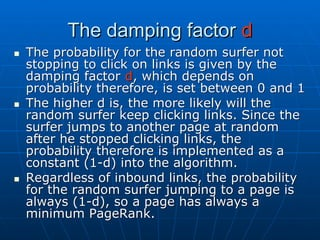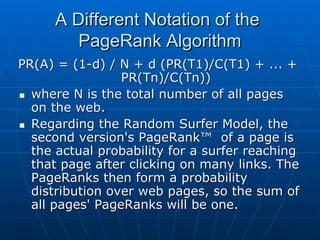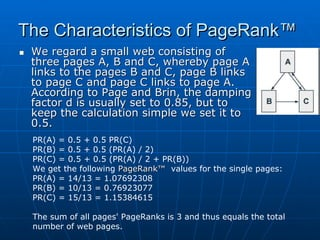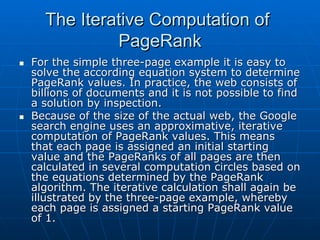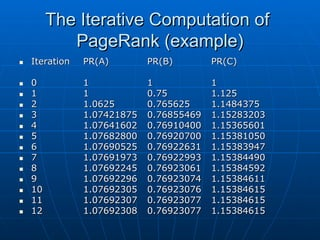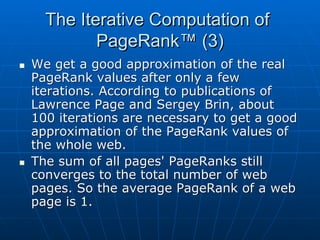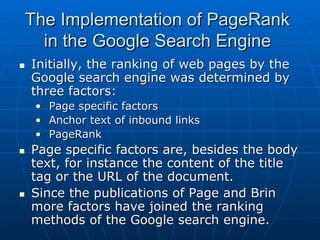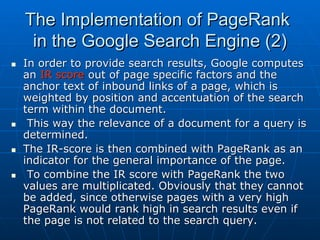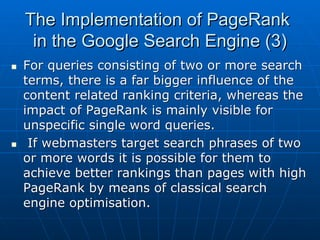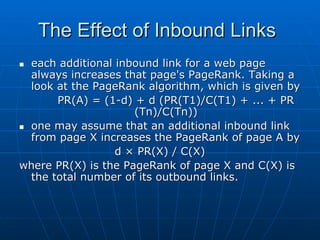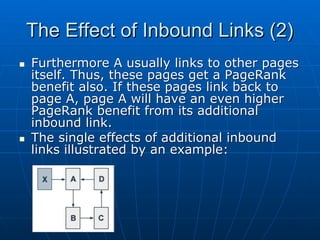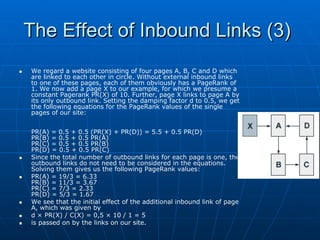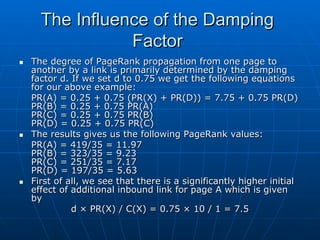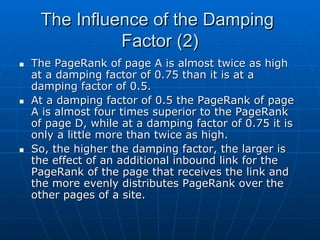1 von 23

### Page rank2

• 1. PageRank™ - Introduction  The heart of Google’s searching software is PageRank™, a system for ranking web pages developed by Larry Page and Sergey Brin at Stanford University
• 2. PageRank™ - Introduction  Essentially, Google interprets a link from page A to page B as a vote, by page A, for page B.  BUT these votes doesn’t weigh the same, because Google also analyzes the page that casts the vote.
• 3. The original PageRank™ algorithm PR(A) = (1-d) + d (PR(T1)/C(T1) + ... + +PR (Tn)/C(Tn)) Where:  PR(A) is the PageRank of page A,  PR(Ti) is the PageRank of pages Ti which link to page A,  C(Ti) is the number of outbound links on page Ti d is a damping factor which can be set between 0 and 1.
• 4. PageRank™ algorithm  It’s obvious that the PageRank™ algorithm does not rank the whole website, but it’s determined for each page individually. Furthermore, the PageRank™ of page A is recursively defined by the PageRank™ of those pages which link to page A
• 5. PR(T1)/C(T1) + ... + PR(Tn)/C(Tn)  The PageRank™ of pages Ti which link to page A does not influence the PageRank™ of page A uniformly.  The PageRank™ of a page T is always weighted by the number of outbound links C(T) on page T.  Which means that the more outbound links a page T has, the less will page A benefit from a link to it on page T.
• 6. PR(T1)/C(T1) + ... + PR(Tn)/C(Tn)  The weighted PageRank™ of pages Ti is then added up. The outcome of this is that an additional inbound link for page A will always increase page A's PageRank™.
• 7. PR(A) = (1-d) + d * (PR(T1)/C(T1) + ... + PR(Tn)/C(Tn))  After all, the sum of the weighted PageRanks of all pages Ti is multiplied with a damping factor d which can be set between 0 and 1. Thereby, the extend of PageRank benefit for a page by another page linking to it is reduced.
• 8. The Random Surfer Model  PageRank™ is considered as a model of user behaviour, where a surfer clicks on links at random with no regard towards content.  The random surfer visits a web page with a certain probability which derives from the page's PageRank.  The probability that the random surfer clicks on one link is solely given by the number of links on that page.  This is why one page's PageRank is not completely passed on to a page it links to, but is devided by the number of links on the page.
• 9. The Random Surfer Model (2)  So, the probability for the random surfer reaching one page is the sum of probabilities for the random surfer following links to this page.  This probability is reduced by the damping factor d. The justification within the Random Surfer Model, therefore, is that the surfer does not click on an infinite number of links, but gets bored sometimes and jumps to another page at random.
• 10. The damping factor d  The probability for the random surfer not stopping to click on links is given by the damping factor d, which depends on probability therefore, is set between 0 and 1  The higher d is, the more likely will the random surfer keep clicking links. Since the surfer jumps to another page at random after he stopped clicking links, the probability therefore is implemented as a constant (1-d) into the algorithm.  Regardless of inbound links, the probability for the random surfer jumping to a page is always (1-d), so a page has always a minimum PageRank.
• 11. A Different Notation of the PageRank Algorithm PR(A) = (1-d) / N + d (PR(T1)/C(T1) + ... + PR(Tn)/C(Tn))  where N is the total number of all pages on the web.  Regarding the Random Surfer Model, the second version's PageRank™ of a page is the actual probability for a surfer reaching that page after clicking on many links. The PageRanks then form a probability distribution over web pages, so the sum of all pages' PageRanks will be one.
• 12. The Characteristics of PageRank™  We regard a small web consisting of three pages A, B and C, whereby page A links to the pages B and C, page B links to page C and page C links to page A. According to Page and Brin, the damping factor d is usually set to 0.85, but to keep the calculation simple we set it to 0.5. PR(A) = 0.5 + 0.5 PR(C) PR(B) = 0.5 + 0.5 (PR(A) / 2) PR(C) = 0.5 + 0.5 (PR(A) / 2 + PR(B)) We get the following PageRank™ values for the single pages: PR(A) = 14/13 = 1.07692308 PR(B) = 10/13 = 0.76923077 PR(C) = 15/13 = 1.15384615 The sum of all pages' PageRanks is 3 and thus equals the total number of web pages.
• 13. The Iterative Computation of PageRank  For the simple three-page example it is easy to solve the according equation system to determine PageRank values. In practice, the web consists of billions of documents and it is not possible to find a solution by inspection.  Because of the size of the actual web, the Google search engine uses an approximative, iterative computation of PageRank values. This means that each page is assigned an initial starting value and the PageRanks of all pages are then calculated in several computation circles based on the equations determined by the PageRank algorithm. The iterative calculation shall again be illustrated by the three-page example, whereby each page is assigned a starting PageRank value of 1.
• 14. The Iterative Computation of PageRank (example)  Iteration PR(A) PR(B) PR(C)  0 1 1 1  1 1 0.75 1.125  2 1.0625 0.765625 1.1484375  3 1.07421875 0.76855469 1.15283203  4 1.07641602 0.76910400 1.15365601  5 1.07682800 0.76920700 1.15381050  6 1.07690525 0.76922631 1.15383947  7 1.07691973 0.76922993 1.15384490  8 1.07692245 0.76923061 1.15384592  9 1.07692296 0.76923074 1.15384611  10 1.07692305 0.76923076 1.15384615  11 1.07692307 0.76923077 1.15384615  12 1.07692308 0.76923077 1.15384615
• 15. The Iterative Computation of PageRank™ (3)  We get a good approximation of the real PageRank values after only a few iterations. According to publications of Lawrence Page and Sergey Brin, about 100 iterations are necessary to get a good approximation of the PageRank values of the whole web.  The sum of all pages' PageRanks still converges to the total number of web pages. So the average PageRank of a web page is 1.
• 16. The Implementation of PageRank in the Google Search Engine  Initially, the ranking of web pages by the Google search engine was determined by three factors: • Page specific factors • Anchor text of inbound links • PageRank  Page specific factors are, besides the body text, for instance the content of the title tag or the URL of the document.  Since the publications of Page and Brin more factors have joined the ranking methods of the Google search engine.
• 17. The Implementation of PageRank in the Google Search Engine (2)  In order to provide search results, Google computes an IR score out of page specific factors and the anchor text of inbound links of a page, which is weighted by position and accentuation of the search term within the document.  This way the relevance of a document for a query is determined.  The IR-score is then combined with PageRank as an indicator for the general importance of the page.  To combine the IR score with PageRank the two values are multiplicated. Obviously that they cannot be added, since otherwise pages with a very high PageRank would rank high in search results even if the page is not related to the search query.
• 18. The Implementation of PageRank in the Google Search Engine (3)  For queries consisting of two or more search terms, there is a far bigger influence of the content related ranking criteria, whereas the impact of PageRank is mainly visible for unspecific single word queries.  If webmasters target search phrases of two or more words it is possible for them to achieve better rankings than pages with high PageRank by means of classical search engine optimisation.
• 19. The Effect of Inbound Links  each additional inbound link for a web page always increases that page's PageRank. Taking a look at the PageRank algorithm, which is given by PR(A) = (1-d) + d (PR(T1)/C(T1) + ... + PR (Tn)/C(Tn))  one may assume that an additional inbound link from page X increases the PageRank of page A by d × PR(X) / C(X) where PR(X) is the PageRank of page X and C(X) is the total number of its outbound links.
• 20. The Effect of Inbound Links (2)  Furthermore A usually links to other pages itself. Thus, these pages get a PageRank benefit also. If these pages link back to page A, page A will have an even higher PageRank benefit from its additional inbound link.  The single effects of additional inbound links illustrated by an example: